Next: Discretization and optimization techniques Up: paper Previous: Introduction

# Necessary conditions for elliptic control problems with control and state constraints

Let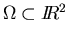be a bounded domain with piecewise smooth boundary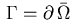. The derivative in the direction of the outward unit normalof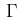will be denoted by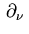. Suppose that the boundary is partioned as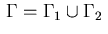with disjoints sets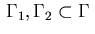that are composed of finitely many smooth and connected components.

We consider the optimal control problem of determining a distributed control function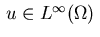that minimizes the functional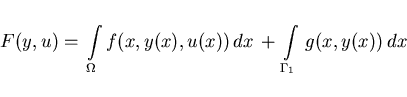(2.1)

subject to the elliptic state equation,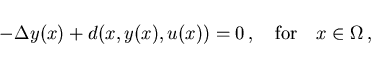(2.2)

Neumann and Dirichlet boundary conditions,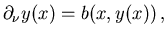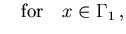(2.3)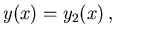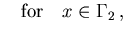(2.4)

and mixed control-state inequality constraints, resp. pure state inequality constraints,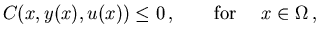(2.5)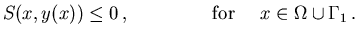(2.6)

The split boundary formulation permits simultaneous treatment of various boundary conditions while in  this was done in separate sections. The functions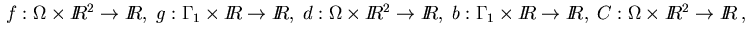and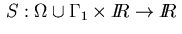are supposed to be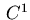-functions and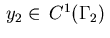is assumed in the Dirichlet condition (2.4). We have to admit that no numerical example with a spliting of the boundary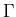into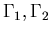will be considered in this paper. However, the spltting has been introduced to allow for a general discussion of necessary conditions. A practical example with splitted boundary may be found in .

The Laplacian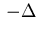in the elliptic equation (2.2) can be replaced by an elliptic operator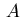in divergence form. We refer to section 2 of  for a precise definition. The above distributed control problem is slightly more general than the one considered in Bonnans and Casas  where first order conditions have been given in terms of a weak and strong Pontryagin principle. For linear elliptic equations, first order conditions may also be found in Bergounioux et al. , Bonnans and Casas . Nonlinear elliptic equations of Lotka-Volterra type have been considered in Cañada et al.  and Leung, Stojanovic [21,28].

Throughout this paper, it wil be assumed that an optimal solution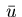and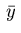of problem (2.1)-(2.6) exists. To ensure well-posedness of the elliptic problem (2.1)-(2.3) we require as in Bonnans, Casas , condition (2.3), that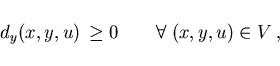(2.7)

holds where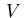is a suitable bounded set containing the graph of the optimal solution. In special cases, such as Examples 1 and 2 below, one can dispense with this condition since well-posedness follows from special results cf. Gunzburger et al. . However, we should note that condition (2.7) is not satisfied for all numerical examples in section 4.

The active sets for the inequality constraints (2.5), (2.6) are given by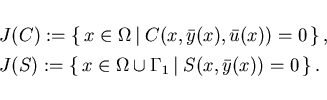(2.8)

We do not study regularity conditions in detail and require that the following ones hold: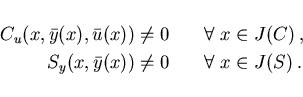(2.9)

Extending in a purely formal way the first order necessary conditions in Bonnans and Casas , we arrive at the following. There exists an adjoint state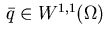, a multiplier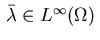, and a regular Borel measure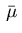in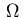such that the following conditions hold: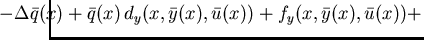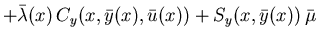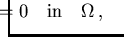(2.10)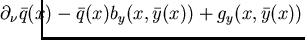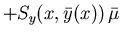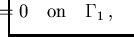(2.11)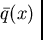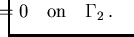(2.12)

minimum condition for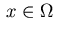: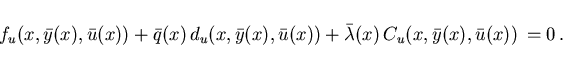(2.13)

complementarity conditions: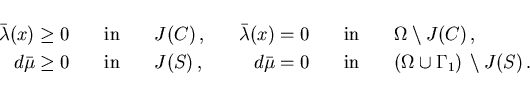(2.14)

The adjoint equations (2.10)-(2.12) are understood in the weak sense. According to Bourbaki , Chapter 9, the regular Borel measure in the adjoint equations (2.10) and (2.11) possesses a decomposition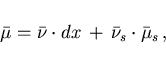(2.15)

where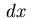represents the Lebesgue measure, the measure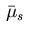is singular with respect toand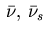are measurable.

In many applications, the control and state constraints (2.5) and (2.6) are simple box constraints of the type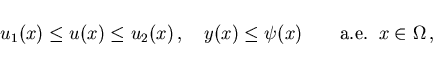(2.16)

with functions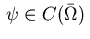and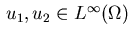. In this case, the adjoint equation (2.10) reduces to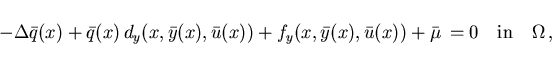(2.17)

while the the minimum condition (2.13) yields the control law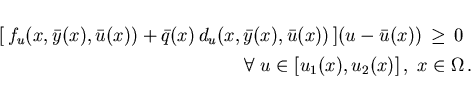(2.18)

A further specialization refers to a cost functional (2.1) of tracking type which has been considered frequently, cf. [1,2,19,20],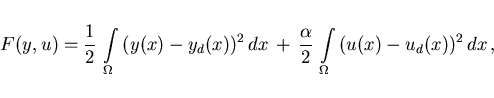(2.19)

with given functions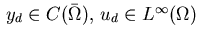, and a nonnegative weight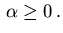Furthermore, let the function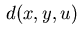in the state equation (2.2) be linear in the control variable with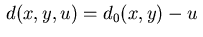. Then we deduce from (2.18) the minimum condition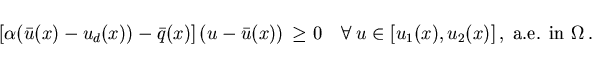(2.20)

Case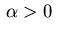: The previous conditions show that the optimal control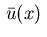is the projection of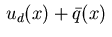onto the interval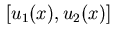. More precisely, we have for: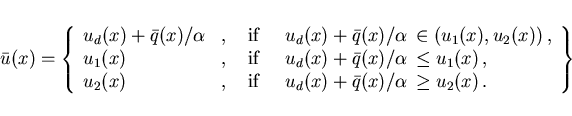(2.21)

Case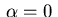: We obtain an optimal control of bang-bang or singular type,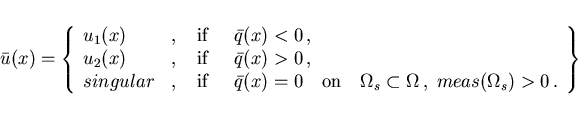(2.22)Next: Discretization and optimization techniques Up: paper Previous: Introduction
Hans D. Mittelmann
2000-10-06